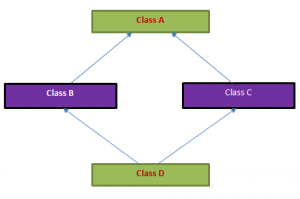# What is the diamond problem and what is the virtual base class in C++?

Let us see at below diagram, which helps us in explaining the diamond problem.In the above picture, we have 2 classes B and C that derive from class – A.  We also have class D that derives from both B and C by using multiple inheritance.
The compiler will get confused that from which base it needs to take.

In the code above, we’ve given a more concrete example of the diamond problem. The MyBaseClass class corresponds to the topmost class in the hierarchy (A in our graphic above), MyDerivedClass and MyDerived2Class respectively correspond to B and C in the graphic, and the MyDerived3Class class corresponds to D.
So, let us look at the output of the above program

In our inheritance hierarchy, we can see that both the MyDerivedClass  and MyDerived2Class classes derive from the MyBaseClass base class.

And here is the problem: because MyDerived3Class derives from both the MyDerivedClass  and MyDerived2Class classes, which each have their own copy of the data members and methods of the MyBaseClass class.

At the end, the MyDerived3Class class object ocClas will contain two object of the MyBaseClass base class.

The compiler gets confused about which copy of the base object is to be taken, so the compiler throws an error that reports as shown above

What is the solution to the Diamond Problem?

Below is the solution for the diamond problem.
If the inheritance from the MyBaseClass class to both the MyDerivedClass and MyDerived2Class class is marked as virtual, then C++ will ensure that only one subobject of the MyBaseClass class will be created for every MyDerived3Class object.
Let us have a look at the example. Copy and compile it.

In the above program, we have derived the base class using the “virtual” keyword to the MyDerivedClass and MyDerived2Class class declarations. Now the MyDerived3Class class object will have only one MyBaseClass sub-object, and the code below will compile just fine, the output of the above program is